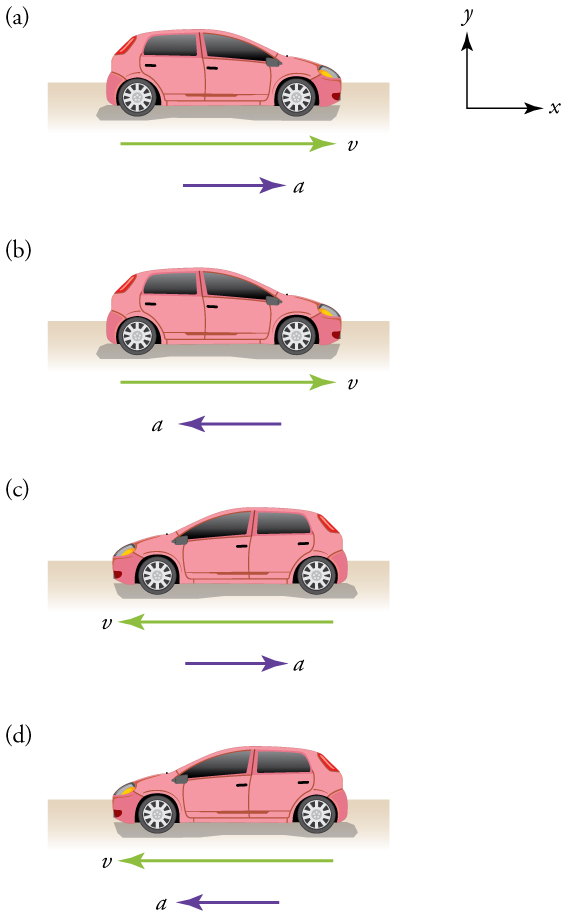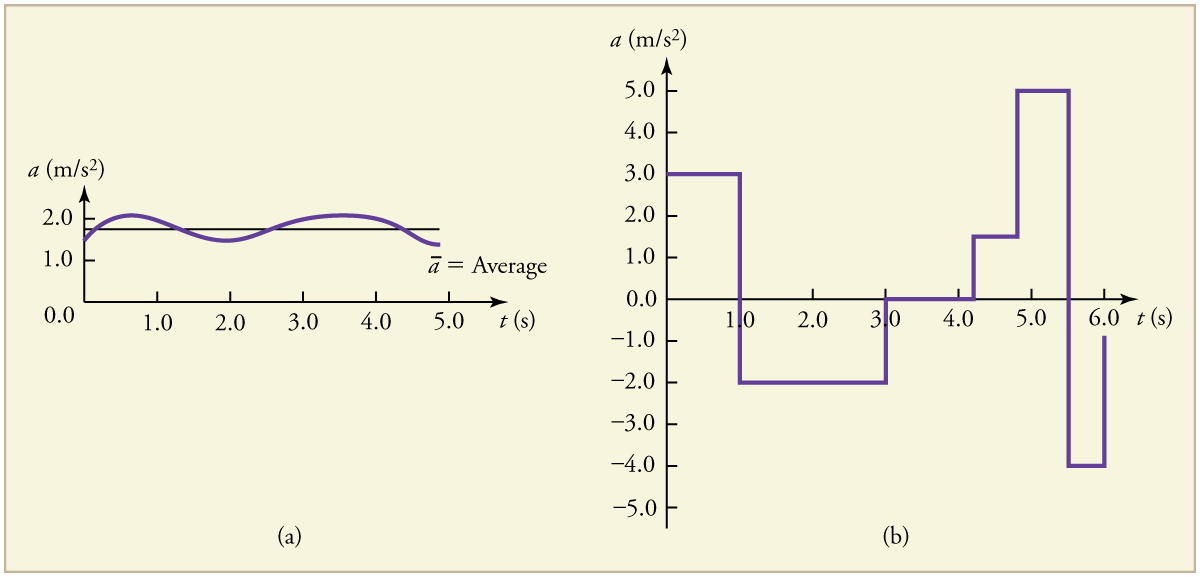# 21 3.4 Acceleration

In everyday conversation, to accelerate means to speed up. Perhaps the most common demonstration of acceleration happens when you press on the gas pedal in your car. The accelerator in a car can in fact cause it to speed up. The greater the acceleration, the greater the change in velocity over a given time. The formal definition of acceleration is consistent with these notions, but more inclusive.

### AVERAGE ACCELERATION

Average acceleration is the rate at which velocity changes,

$\overrightarrow{\mathbf{a}}_{average} = \frac{\Delta\overrightarrow{\mathbf{v}}}{\Delta\mathbf{t}} = \frac{\overrightarrow{\mathbf{v_f}}-\overrightarrow{\mathbf{v_i}}}{\mathbf{t_f-t_i}}$

where $\overrightarrow{\mathbf{a}}$ is average acceleration, $\overrightarrow{\mathbf{v}}$ is velocity, and t is time.

Because acceleration is velocity in m/s divided by time in s, the standard units for acceleration are m/s2, meters per second squared or meters per second per second, which literally means by how many meters per second the velocity changes every second.

Recall that velocity is a vector—it has both magnitude and direction. This means that a change in velocity can be a change in magnitude (or speed), but it can also be a change in direction. For example, if a cyclist turns a corner at constant speed, it is accelerating because its direction is changing. The quicker you turn, the greater the acceleration. So there is an acceleration when velocity changes either in magnitude (an increase or decrease in speed) or in direction, or both.

### ACCELERATION AS A VECTOR

Acceleration is a vector in the same direction as the change in velocity, $\Delta\overrightarrow{\mathbf{v}}$. Since velocity is a vector, it can change either in magnitude or in direction. Acceleration is therefore a change in either speed or direction, or both.

Keep in mind that although acceleration is in the direction of the change in velocity, it is not always in the direction of motion. When an object slows down, its acceleration is opposite to the direction of its motion. This is known as deceleration.

### MISCONCEPTION ALERT: DECELERATION VS. NEGATIVE ACCELERATION

Deceleration always refers to acceleration in the direction opposite to the direction of the velocity. Deceleration always reduces speed. Negative acceleration, however, is acceleration in the negative direction in the chosen coordinate system. Negative acceleration may or may not be deceleration, and deceleration may or may not be considered negative acceleration. For example, consider the car shown below here:Figure 3. (a) This car is speeding up as it moves toward the right. It therefore has positive acceleration in our coordinate system. (b) This car is slowing down as it moves toward the right. Therefore, it has negative acceleration in our coordinate system, because its acceleration is toward the left. The car is also decelerating: the direction of its acceleration is opposite to its direction of motion. (c) This car is moving toward the left, but slowing down over time. Therefore, its acceleration is positive in our coordinate system because it is toward the right. However, the car is decelerating because its acceleration is opposite to its motion. (d) This car is speeding up as it moves toward the left. It has negative acceleration because it is accelerating toward the left. However, because its acceleration is in the same direction as its motion, it is speeding up (not decelerating).

### Example 1: Calculating Acceleration: A Racehorse Leaves the Gate

A racehorse coming out of the gate accelerates from rest to a velocity of 15.0 m/s due west in 1.80 s. What is its average acceleration?

Strategy

First we draw a sketch and assign a coordinate system to the problem. This is a simple problem, but it always helps to visualize it. Notice that we assign east as positive and west as negative. Thus, in this case, we have negative velocity.

We can solve this problem by identifying $\Delta\overrightarrow{\mathbf{v}}$ and Δt from the given information and then calculating the average acceleration directly from the equation:

$\overrightarrow{\mathbf{a}}_{average} = \frac{\Delta\overrightarrow{\mathbf{v}}}{\Delta\mathbf{t}} = \frac{\overrightarrow{\mathbf{v_f}}-\overrightarrow{\mathbf{v_i}}}{\mathbf{t_f-t_i}}$

Solution

1. Identify the knowns $\overrightarrow{\mathbf{v_i}}=0\textbf{ m/s}$, $\overrightarrow{\mathbf{v_f}}=-15 \textbf{ m/s}$, (the negative sign indicates direction toward the west), Δt = 1.80 s.

2. Find the change in velocity. Since the horse is going from zero to -15.0 m/s, its change in velocity equals its final velocity: $\Delta\overrightarrow{\mathbf{v}} = \overrightarrow{\mathbf{v_f}} = -15.0 \textbf{m/s}$.

3. Plug in the known values ($\Delta\overrightarrow{\mathbf{v}}$ and Δt) and solve for the unknown $\overrightarrow{\mathbf{a}}$.

$\overrightarrow{\mathbf{a}}_{average} = \frac{\Delta\overrightarrow{\mathbf{v}}}{\Delta\mathbf{t}} = \frac{\overrightarrow{\mathbf{v_f}}-\overrightarrow{\mathbf{v_i}}}{\mathbf{t_f-t_i}} = \frac{(-15 \textbf{ m/s}-0 \textbf{ m/s})}{1.80 \textbf{ s}}=-8.33\textbf{ }\mathbf{ m/s^2}$

Discussion

The negative sign for acceleration indicates that acceleration is toward the west. An acceleration of 8.33 m/s2 due west means that the horse increases its velocity by 8.33 m/s due west each second, that is, 8.33 meters per second per second, which we write as 8.33 m/s2. This is truly an average acceleration, because the ride is not smooth. We shall see later that an acceleration of this magnitude would require the rider to hang on with a force nearly equal to his weight.

# Instantaneous Acceleration

Instantaneous acceleration, $\overrightarrow{\mathbf{a}}$, or the acceleration at a specific instant in time, is obtained by the same process as discussed for instantaneous velocity in the earlier section on speed and velocity —that is, by considering an infinitesimally small interval of time. How do we find instantaneous acceleration using only algebra? The answer is that we choose an average acceleration that is representative of the motion. Figure 6 below hows graphs of instantaneous acceleration versus time for two very different motions. In Figure 6 a), the acceleration varies slightly and the average over the entire interval is nearly the same as the instantaneous acceleration at any time. In this case, we should treat this motion as if it had a constant acceleration equal to the average (in this case about 1.8 m/s2). In Figure 6 b), the acceleration varies drastically over time. In such situations it is best to consider smaller time intervals and choose an average acceleration for each. For example, we could consider motion over the time intervals from 0 to 1.0 s and from 1.0 to 3.0 s as separate motions with accelerations of +3.0 m/s2 and -2.0 m/s2, respectively.Figure 6. Graphs of instantaneous acceleration versus time for two different one-dimensional motions. (a) Here acceleration varies only slightly and is always in the same direction, since it is positive. The average over the interval is nearly the same as the acceleration at any given time. (b) Here the acceleration varies greatly, perhaps representing a package on a post office conveyor belt that is accelerated forward and backward as it bumps along. It is necessary to consider small time intervals (such as from 0 to 1.0 s) with constant or nearly constant acceleration in such a situation.

Perhaps the most important thing to note about these examples is the signs of the answers. In our chosen coordinate system, plus means the quantity is to the right and minus means it is to the left. This is easy to imagine for displacement and velocity. But it is a little less obvious for acceleration. Most people interpret negative acceleration as the slowing of an object. The crucial distinction if the acceleration is in the opposite direction from the velocity. In fact, a negative acceleration will increase a negative velocity. If acceleration has the same sign as the velocity, the object is speeding up. If acceleration has the opposite sign as the velocity, the object is slowing down.

### PHET EXPLORATIONS: MOVING MAN SIMULATION

Learn about position, velocity, and acceleration graphs. Move the little man back and forth with the mouse and plot his motion. Set the position, velocity, or acceleration and let the simulation move the man for you.

# Section Summary

• Acceleration is the rate at which velocity changes. In symbols, average acceleration is
$\overrightarrow{\mathbf{a}}_{average} = \frac{\Delta\overrightarrow{\mathbf{v}}}{\Delta\mathbf{t}} = \frac{\overrightarrow{\mathbf{v_f}}-\overrightarrow{\mathbf{v_i}}}{\mathbf{t_f-t_i}}$
• The standard unit for acceleration is m/s2.
• Acceleration is a vector, and thus has a both a magnitude and direction.
• Acceleration can be caused by either a change in the magnitude or the direction of the velocity.
• Instantaneous acceleration, $\overrightarrow{\mathbf{a}}$, is the acceleration at a specific instant in time.
• Deceleration is an acceleration with a direction opposite to that of the velocity.

## Glossary

acceleration
the rate of change in velocity; the change in velocity over time
average acceleration
the change in velocity divided by the time over which it changes
instantaneous acceleration
acceleration at a specific point in time
deceleration
acceleration in the direction opposite to velocity; acceleration that results in a decrease in velocity